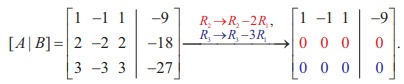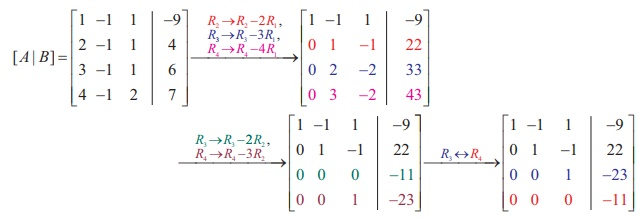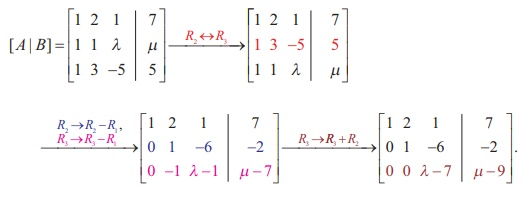Home | | Maths 12th Std | Matrix: Non-homogeneous Linear Equations

# Matrix: Non-homogeneous Linear Equations

Applications of Matrices: Consistency of System of Linear Equations by Rank Method

## Applications of Matrices: Consistency of System of Linear Equations by Rank Method

In second previous section, we have already defined consistency of a system of linear equation. In this section, we investigate it by using rank method. We state the following theorem without proof:

## Theorem 1.14 (Rouch├ę - Capelli Theorem)

A system of linear equations, written in the matrix form as AX = B, is consistent if and only if the rank of the coefficient matrix is equal to the rank of the augmented matrix; that is, ¤ü A= ¤ü ([ B]).

We apply the theorem in the following examples.

Non-homogeneous Linear Equations

Example 1.29

Test for consistency of the following system of linear equations and if possible solve:

+ 2 Ôłĺ = 3, 3Ôłĺ + 2= 1, Ôłĺ 2 + 3= 3, Ôłĺ +1 = 0 .

Solution

Here the number of unknowns is 3.

The matrix form of the system is AX = B, whereApplying Gaussian elimination method on [ A | B], we getThere are three non-zero rows in the row-echelon form of [A | B].So, ¤ü ([A | B] ) . = 3

So, the row-echelon form of A is. There are three non-zero rows in it. So ¤ü(A) = 3.

Hence, ¤ü(A) = ¤ü([ A | B ]) = 3.

From the echelon form, we write the equivalent system of equations

x + 2y ÔÇôz =3, 7y-5z = 8, z=4, 0=0.

The last equation 0 = 0 is meaningful. By the method of back substitution, we get

z = 4

7y - 20 = 8 Ôçĺ y = 4 ,

x = 3 - 8 + 4 Ôçĺ x = Ôłĺ1.

So, the solution is (x = Ôłĺ1, y = 4,  z = 4) .(Note that A is not a square matrix.)

Here the given system is consistent and the solution is unique.

Example 1.30

Test for consistency of the following system of linear equations and if possible solve:

4Ôłĺ 2 + 6= 8, Ôłĺ 3= Ôłĺ1, 15Ôłĺ 3+ 9= 21.

Solution

Here the number of unknowns is 3.

The matrix form of the system is AX B, whereApplying elementary row operations on the augmented matrix[ B], we getSo, ¤ü A) = ¤ü ([ B]) = 2 < 3. From the echelon form, we get the equivalent equations

- 3z  =  -1, - 3= -2 , 0 = 0 .

The equivalent system has two non-trivial equations and three unknowns. So, one of the unknowns should be fixed at our choice in order to get two equations for the other two unknowns. We fix arbitrarily as a real number  , and we get 3- 2, -1- (3- 2) + 31. So, the solution is ( x =1, y = 3t - 2, z = t ), where t is real . The above solution set is a one-parameter family of solutions.

Here, the given system is consistent and has infinitely many solutions which form a one parameter family of solutions.

Note

In the above example, the square matrix A is singular and so matrix inversion method cannot be applied to solve the system of equations. However,Gaussian elimination method is applicable and we are able to decide whether the system is consistent or not. The next example also confirms the supremacy of Gaussian elimination method over other methods.

Example 1.31

Test for consistency of the following system of linear equations and if possible solve:

Ôłĺ = Ôłĺ9, 2Ôłĺ 2 + 2= Ôłĺ18, 3Ôłĺ 3+ 3+ 27 = 0.

Solution

Here the number of unknowns is 3.

The matrix form of the system is AX B, whereApplying elementary row operations on the augmented matrix[ B], we getSo, ¤ü A) = ¤ü ([ B]) = 1 < 3.

From the echelon form, we get the equivalent equations x - y + z = -9, 0 = 0, 0 = 0.

The equivalent system has one non-trivial equation and three unknowns.

Taking y = s, z = t arbitrarily, we get x - s + t = -9; or x = -9 + s - t.

So, the solution is ( = -9 + ts)where and are parameters.

The above solution set is a two-parameter family of solutions.

Here, the given system of equations is consistent and has infinitely many solutions which form a two parameter family of solutions.

Example 1.32

Test the consistency of the following system of linear equations

Ôłĺ = Ôłĺ9, 2Ôłĺ = 4, 3Ôłĺ = 6, 4Ôłĺ + 2= 7.

Solution

Here the number of unknowns is 3.

The matrix form of the system of equations is AX B, whereApplying elementary row operations on the augmented matrix [A|B], we getSo, ¤ü (A) = 3 and ¤ü ([ A | B]) = 4. Hence ¤ü ( A) Ôëá ¤ü ([ A | B]).

If we write the equivalent system of equations using the echelon form, we get

x - y + z = -9, = 22, -23, 0 = -11.

The last equation is a contradiction.

So the given system of equations is inconsistent and has no solution. By Rouch├ę - Capelli theorem, we have the following rule:

┬Ě        If there are n unknowns in the system of equations and ¤ü ( A) = ¤ü ([ A | B]) = n,  then the system AX Bis consistent and has a unique solution.

┬Ě        If there are unknowns in the system AX Band ¤ü A¤ü ([ B]) = kÔëá 0 then the system  is  consistent  and  has  infinitely  many  solutions  and  these  solutions  form  a k - parameter family. In particular, if there are 3 unknowns in a system of equations and ¤ü ( A) = ¤ü ([A | B]) = 2, then the system has infinitely many solutions and these solutions form a one parameter family. In the same manner, if there are 3unknowns in a system of equations and  ¤ü ( A) = ¤ü ([ A | B]) = 1, then the system has infinitely many solutions and these solutions form a two parameter family.

┬Ě        If ¤ü AÔëá ¤ü ([ B]), then the system AX is inconsistent and has no solution.

### Example 1.33

Find the condition on a, b and c so that the following system of linear equations has one parameter family of solutions: x + y + z = a, x + 2 y + 3z = b, 3x + 5 y + 7z = c.

Solution

Here the number of unknowns is 3.

The matrix form of the system is AX B, whereApplying elementary row operations on the augmented matrix [ B], we getIn order that the system should have one parameter family of solutions, we must have ¤ü A) = ¤ü ([ AB]) = 2. So, the third row in the echelon form should be a zero row.

So, - 2= 0 Ôçĺ + 2b.

### Example 1.34

Investigate for what values of ╬╗ and ╬╝ the system of linear equations

+ 2 = 7, ╬╗ z ╬╝+ 3Ôłĺ 5= 5 has

(i) no solution (ii) a unique solution (iii) an infinite number of solutions.

### Solution

Here the number of unknowns is 3.

The matrix form of the system is AX B, whereApplying elementary row operations on the augmented matrix [ B], we get(i) If  ╬╗ = 7 and ╬╝ Ôëá 9 , then ¤ü (A) = 2 and ¤ü ([ A | B]) = 3. So ¤ü ( A) Ôëá ¤ü ([ A | B]). Hence the given system is inconsistent and has no solution.

(ii)    If  ╬╗ Ôëá 7 and m is any real number, then ¤ü (A) = 3 and ¤ü ([ A | B]) = 3.

So ¤ü (A) = ¤ü ([ A | B]) = 3 = Number of unknowns. Hence the given system is consistent and has a unique solution.

(iii) If  ╬╗ = 7 and ╬╝ = 9, then ¤ü(A) = 2 and ¤ü ([ A | B]) = 2.

So, ¤ü(A) = ¤ü ([ A | B]) = 2 < Number of unknowns. Hence the given system is consistent and has infinite number of solutions.

Tags : Definition, Theorem, Formulas, Solved Example Problems | Applications of Matrices: Consistency of System of Linear Equations by Rank Method , 12th Mathematics : UNIT 1 : Applications of Matrices and Determinants
Study Material, Lecturing Notes, Assignment, Reference, Wiki description explanation, brief detail
12th Mathematics : UNIT 1 : Applications of Matrices and Determinants : Matrix: Non-homogeneous Linear Equations | Definition, Theorem, Formulas, Solved Example Problems | Applications of Matrices: Consistency of System of Linear Equations by Rank Method